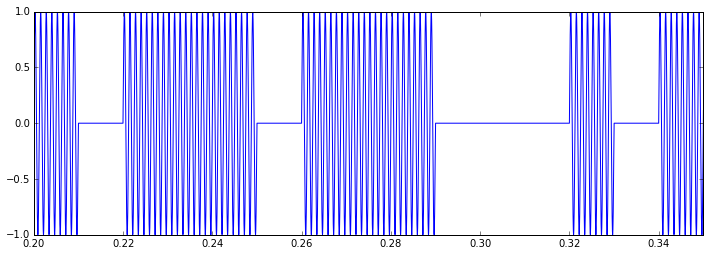In :
%matplotlib inline
import math
import random
random.seed(0)

import matplotlib.pyplot as plt
from IPython.display import Audio
import requests

API_URL = 'http://simphone.herokuapp.com/json'


# On-Off Keying (OOK) Modulation/Demodulation Demo¶

To pass a digital signal over a fake phone line, we need to modulate it. Phone lines are designed to just-barely carry speech by transmitting 300 to 3400 Hz signals. By reducing the bandwidth (frequency, not data) to where speech is just barely intelligible, phone companies can save you money!

Anyways, because the phone line doesn't pass baseband (DC) signals, we need to modulate the signal, i.e. turn it into sound. The laziest way to do so is to play a tone (be on) when there's a 1, and nothing (be off) when there's 0. This is on-off keying.

### Protocol Definition¶

Things that are known to both the modulator and demodulator so they can talk.

In :
FS = 44100 # Hz, sample rate

BAUD = 100 # symbol rate
SYMBOLS = 2 # binary
FCARRIER = 800 # Hz

APPROX_TX_TIME = 3.9 # seconds
SYMBOLS_TO_TX = int(BAUD * APPROX_TX_TIME)
TX_TIME = SYMBOLS_TO_TX / BAUD # after rounding


### Some Data to Transmit¶

In :
data = [random.randrange(SYMBOLS) for n in range(SYMBOLS_TO_TX)]
print(''.join(str(x) for x in data))

110111111001001010011011101110001011010000010011011010110110100001100000011001111101011000100101100000000001010000001000101000111001001011100000110100111111001010100111101110001000111011001010110011110100001110000000011001100110101001000011101111001100011110100100111010100100111110011110101101100100000010011110101010101000100111000000111001011111100011100101110000000101100111000101111000


## Modulating¶

In :
t = list(n/FS for n in range(int(FS * TX_TIME)))
modulator = [data[int(tp * BAUD)] for tp in t]

carrier = [math.sin(2 * math.pi * FCARRIER * tp) for tp in t]

signal = [m * c for m, c in zip(modulator, carrier)]

In :
def pltsetup(bounds):
f, ax = plt.subplots()
f.set_size_inches((12, 4))
ax.set_xlim(bounds)
return f, ax

f, ax = pltsetup((0.2, 0.35))
ax.plot(t, signal)

Out:
[<matplotlib.lines.Line2D at 0x6ec43c8>]In :
print('Transmit file is {:.3f} seconds in length.'.format(len(signal) / FS))
Audio(signal, rate=FS)

Transmit file is 3.900 seconds in length.

Out:

## Processing¶

To filter and add noise to the audio, we'll pass it to the web API. We simply POST an object with a data key (and optional seed and snr attributes)

In :
request = {
'data': signal,
'seed': 24601,
'snr': 5, # default 30
}

In :
received = reply['data']

In :
Audio(received, rate=FS)

Out:
In :
f, ax = pltsetup((0.2, 0.35))

[<matplotlib.lines.Line2D at 0x89042e8>]